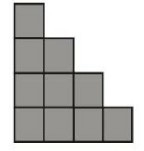(1) $32 \mathrm{~cm}$
(2) $28 \mathrm{~cm}$
(3) $24 \mathrm{~cm}$
(4) $36 \mathrm{~cm}$"
">

# The given figure is made up of 10 squares of the same size. The area of the figure is $40 \mathrm{~cm}^{2}$. Find the perimeter of the figure.(1) $32 \mathrm{~cm}$(2) $28 \mathrm{~cm}$(3) $24 \mathrm{~cm}$(4) $36 \mathrm{~cm}$"

Given:

The area of the figure is $40 \mathrm{~cm}^{2}$.

To do:

We have to find the perimeter of the figure.

Solution:

Let the side of each square be $x$.

This implies,

Area of each square$=x^2$

Area of 10 squares$=10\times x^2=10x^2$  Therefore,

$10x^2=40\ cm^2$

$x^2=\frac{40}{10}$

$x^2=4\ cm^2$

$x=\sqrt{4}\ cm$

$x=2\ cm$

The perimeter of the given figure is the sum of the outer edges of the figure.

Perimeter of the given figure$=16\times2\ cm=32\ cm$.

The correct option is (1) $32\ cm$.

Updated on: 10-Oct-2022

35 Views